# Maharashtra (MSBSHSE) SSC Board Question Paper For Class 10 Maths Paper 1 (Geometry) 2016 In PDF

## Maharashtra SSC Maths Geometry (Paper 1) Exam Question Paper 2016 with Solutions – Free Download

MSBSHSE (Maharashtra board) Annual Exam Class 10 Maths 2016 Geometry Question Paper with solutions are available at BYJU’S, which can be accessed in a pdf format and can also be downloaded easily. Maharashtra board has two papers in each year of the SSC Class 10 Maths exam, which comprise all the concepts of Class 10 Maths, segregated into two parts, namely Algebra and Geometry. Geometry is very important for the students, since it has applications in everyday life. These concepts are useful for the students in their higher classes. Students can get Msbshse ssc Class 10 Maths board previous year question papers as well from BYJU’S.

### MSBSHSE Class 10 Mathematics Question Paper 2016 Geometry Paper with Solutions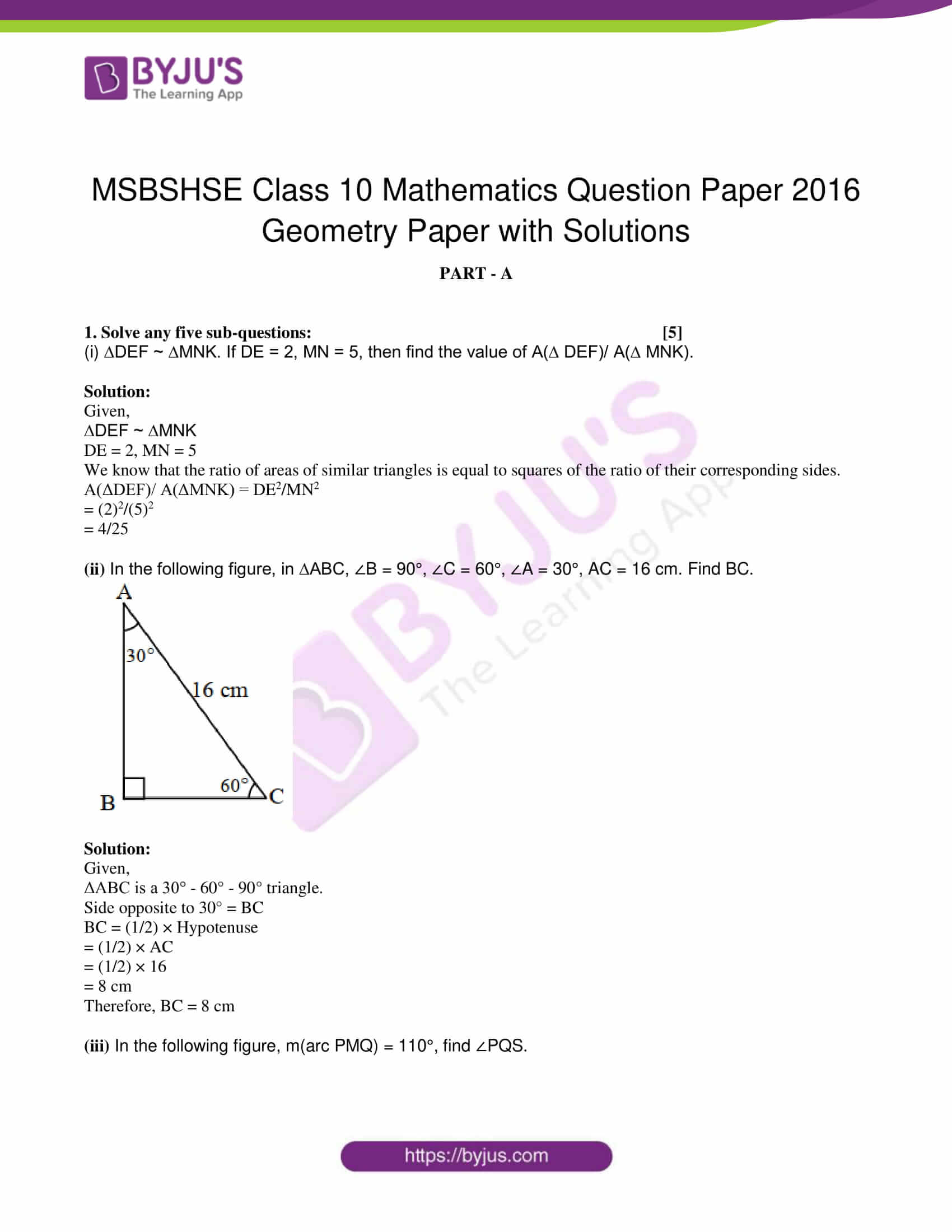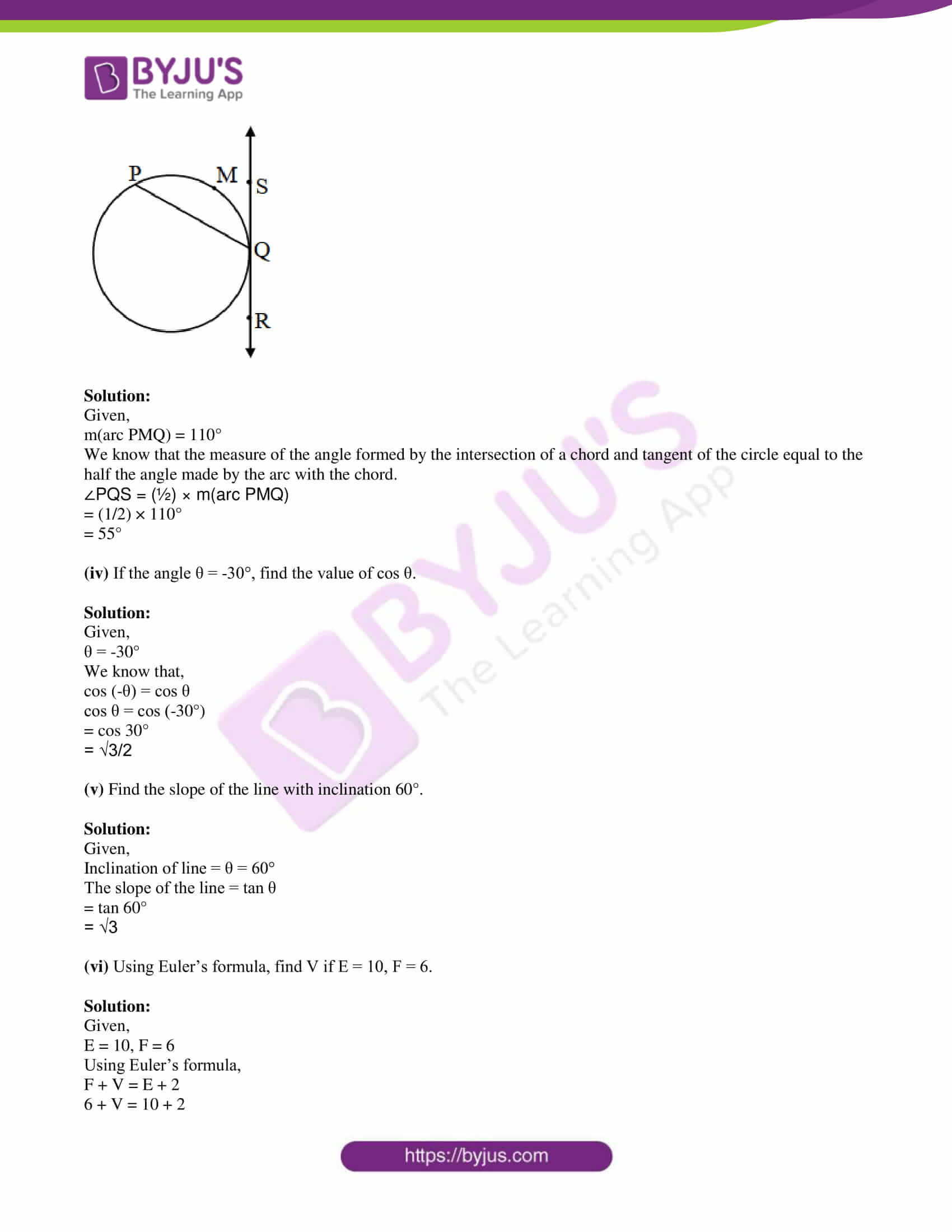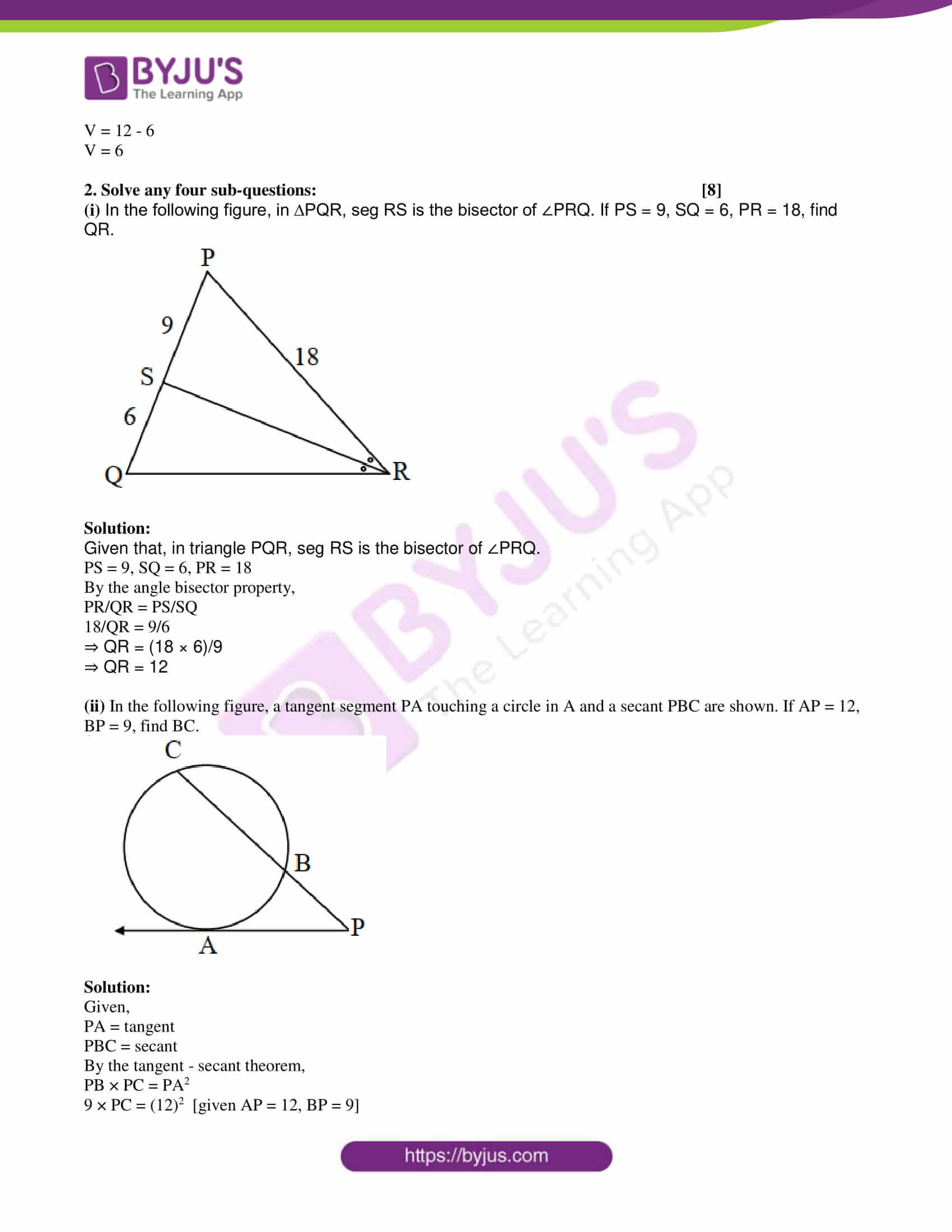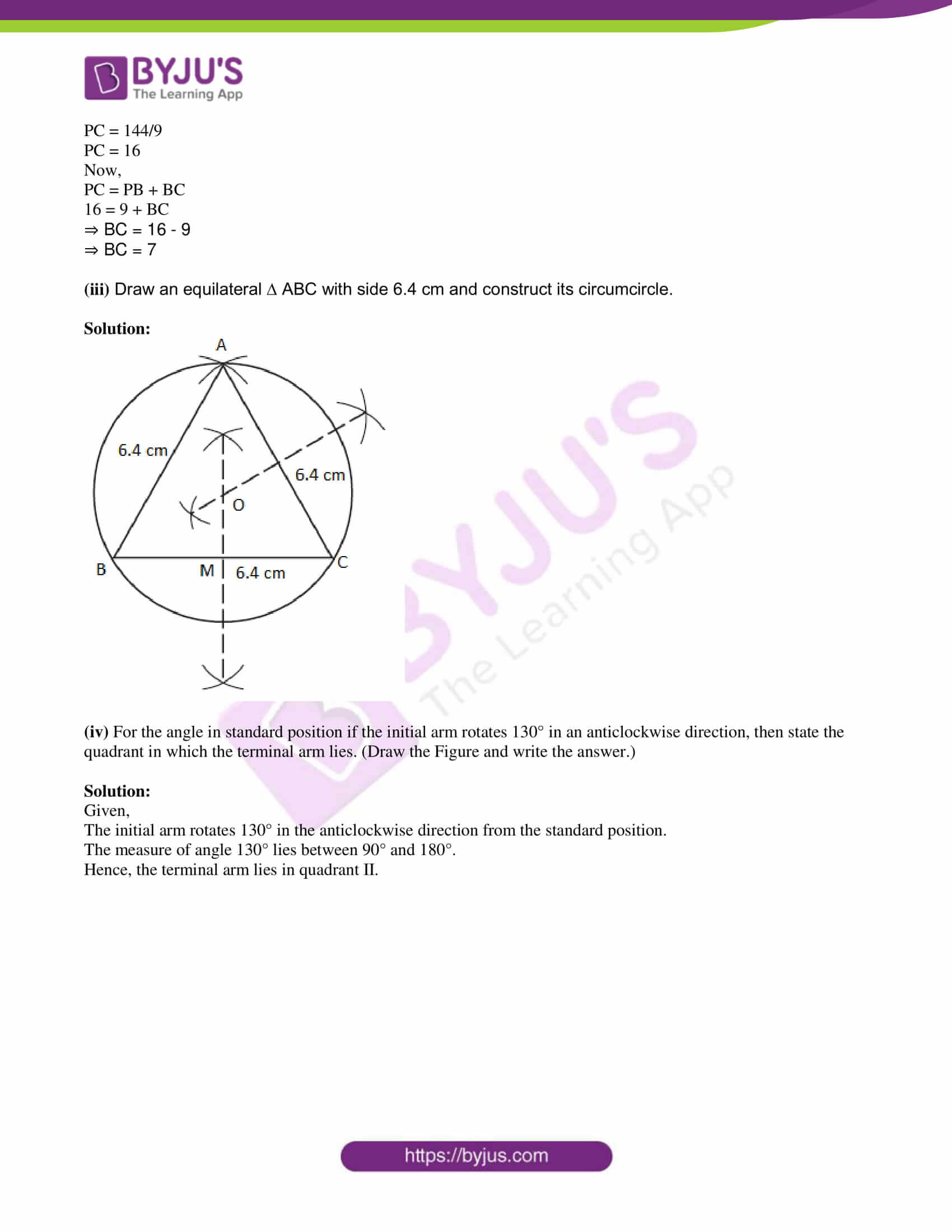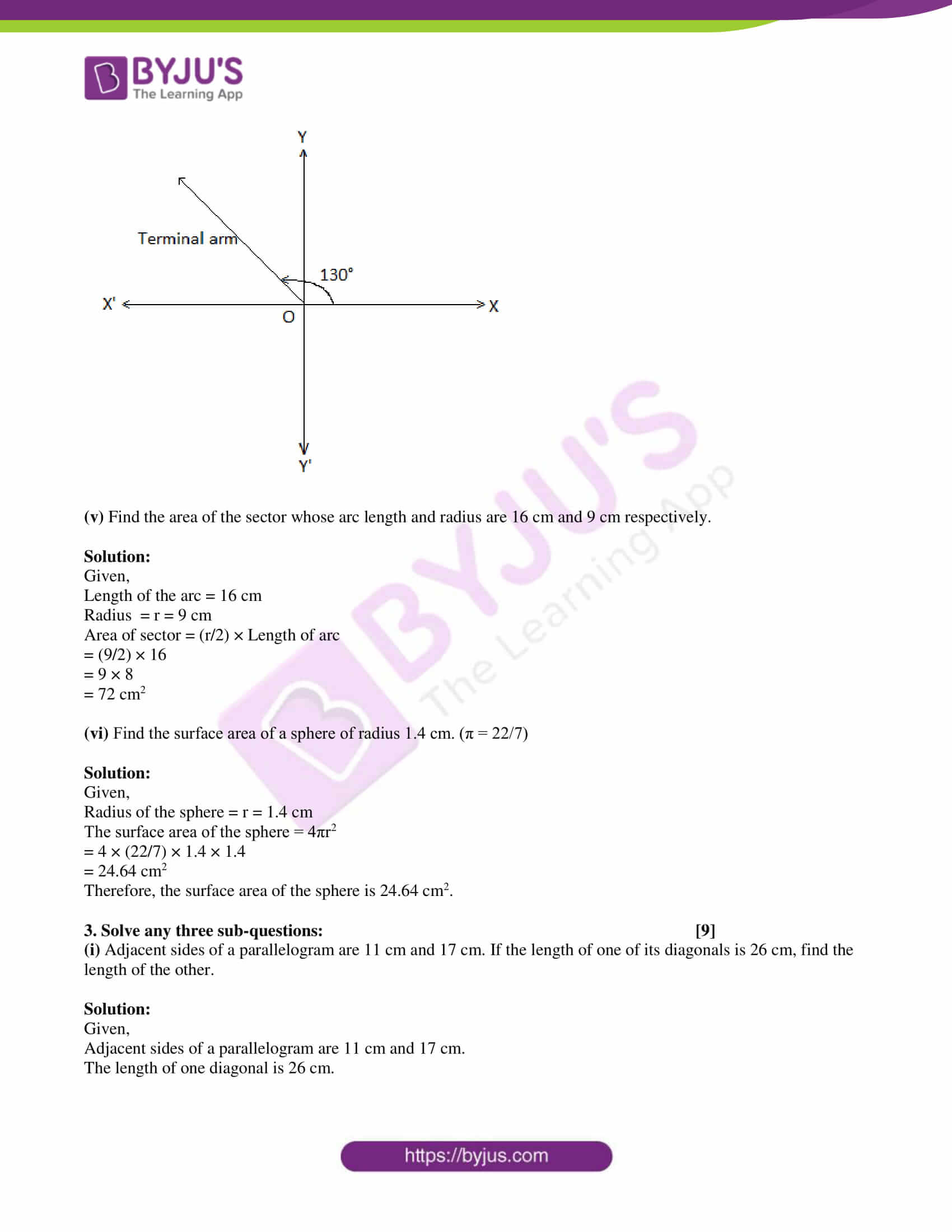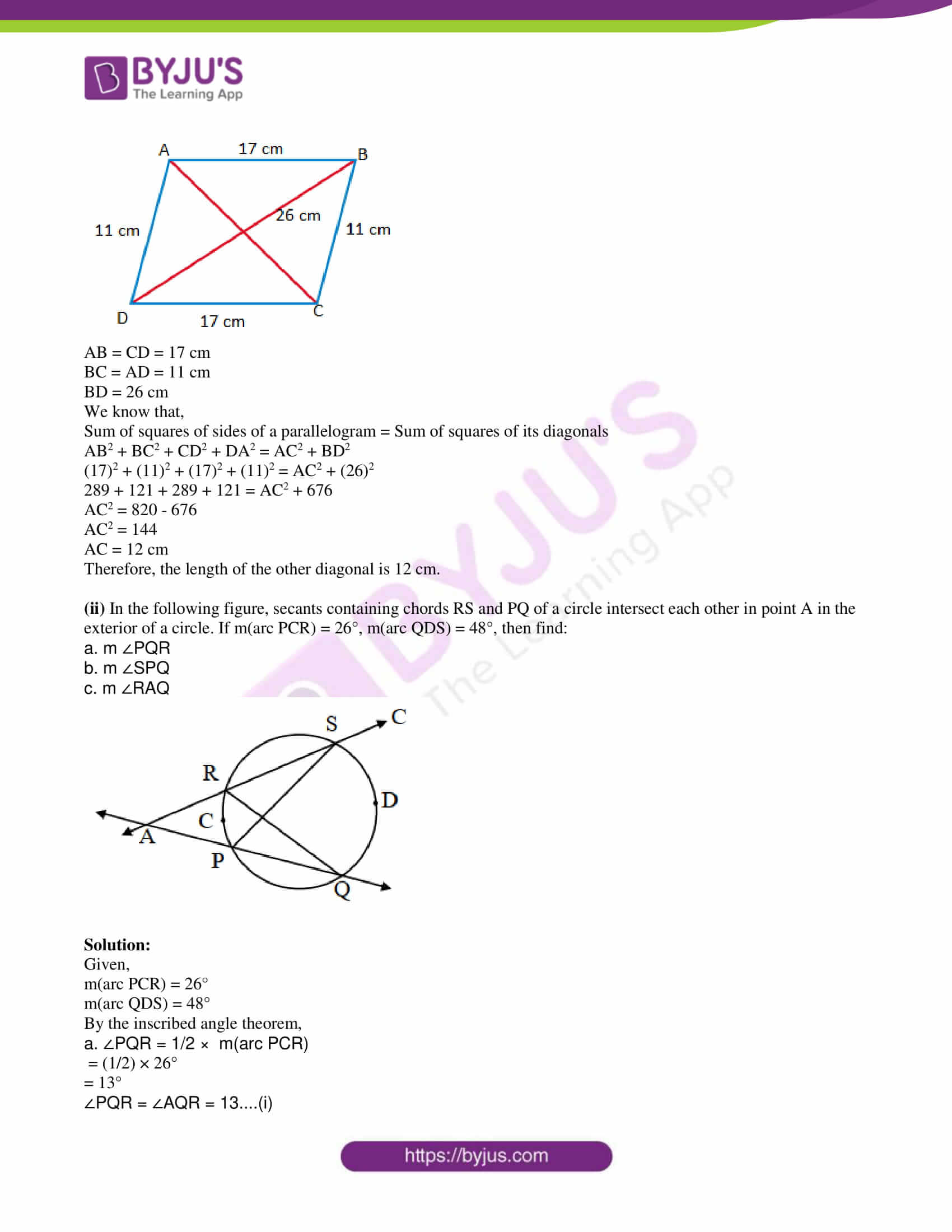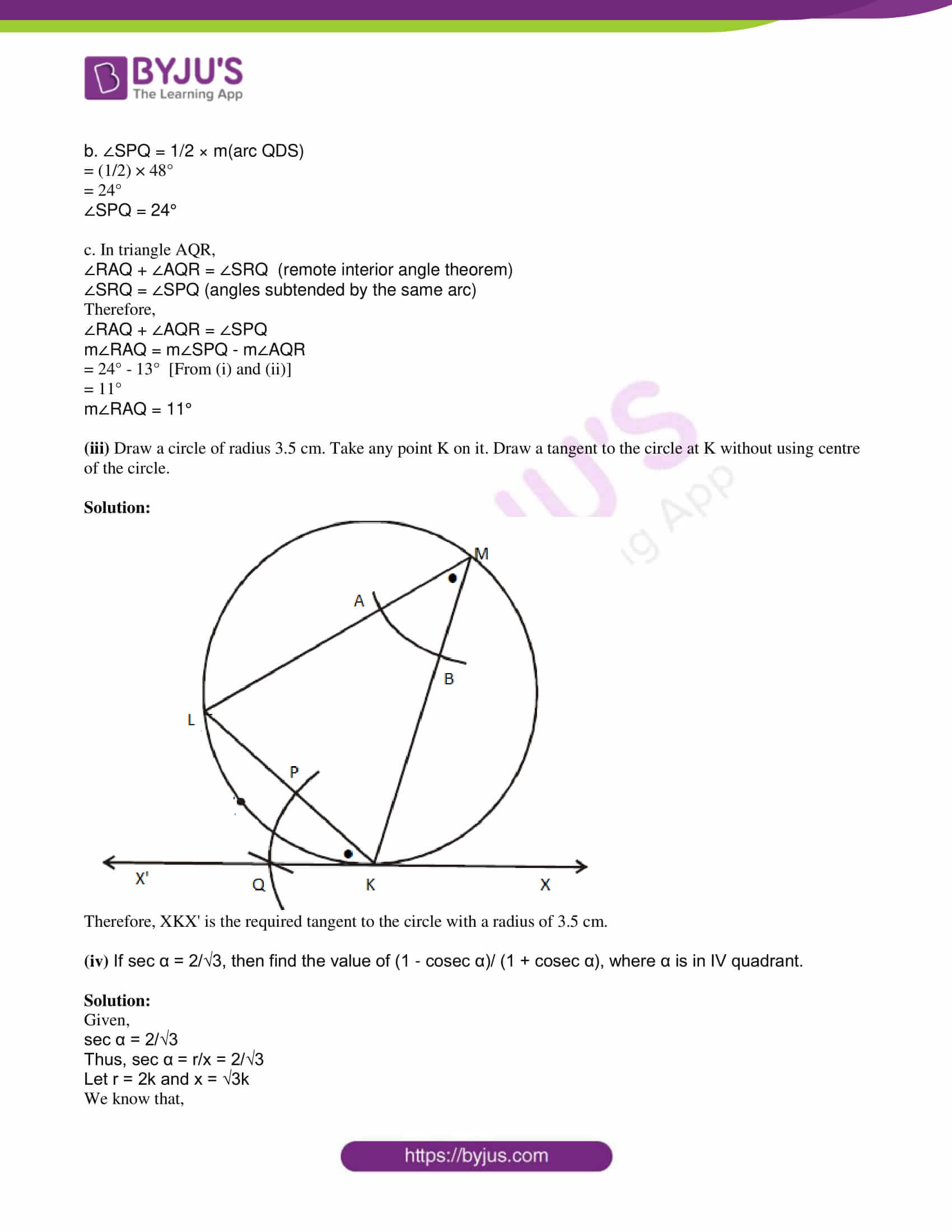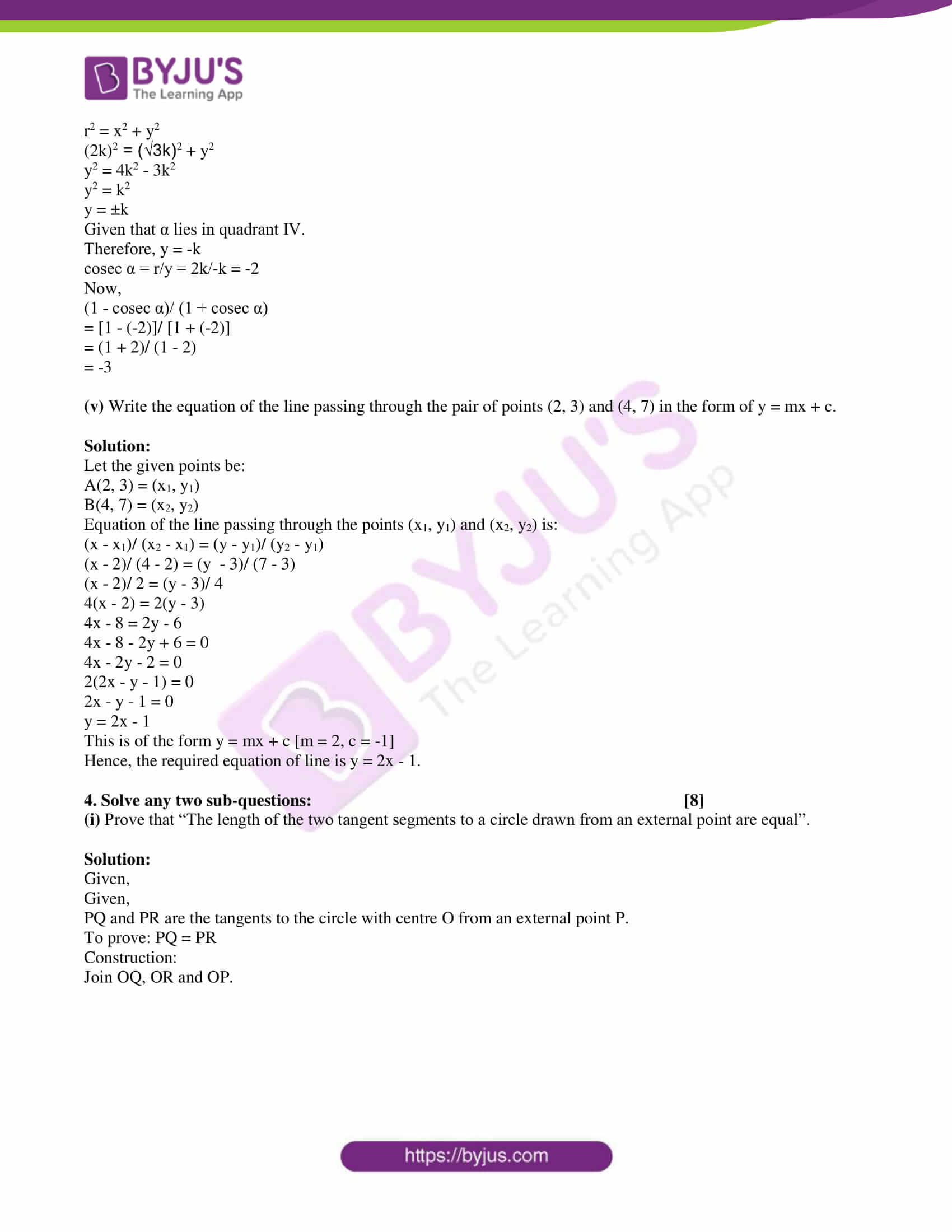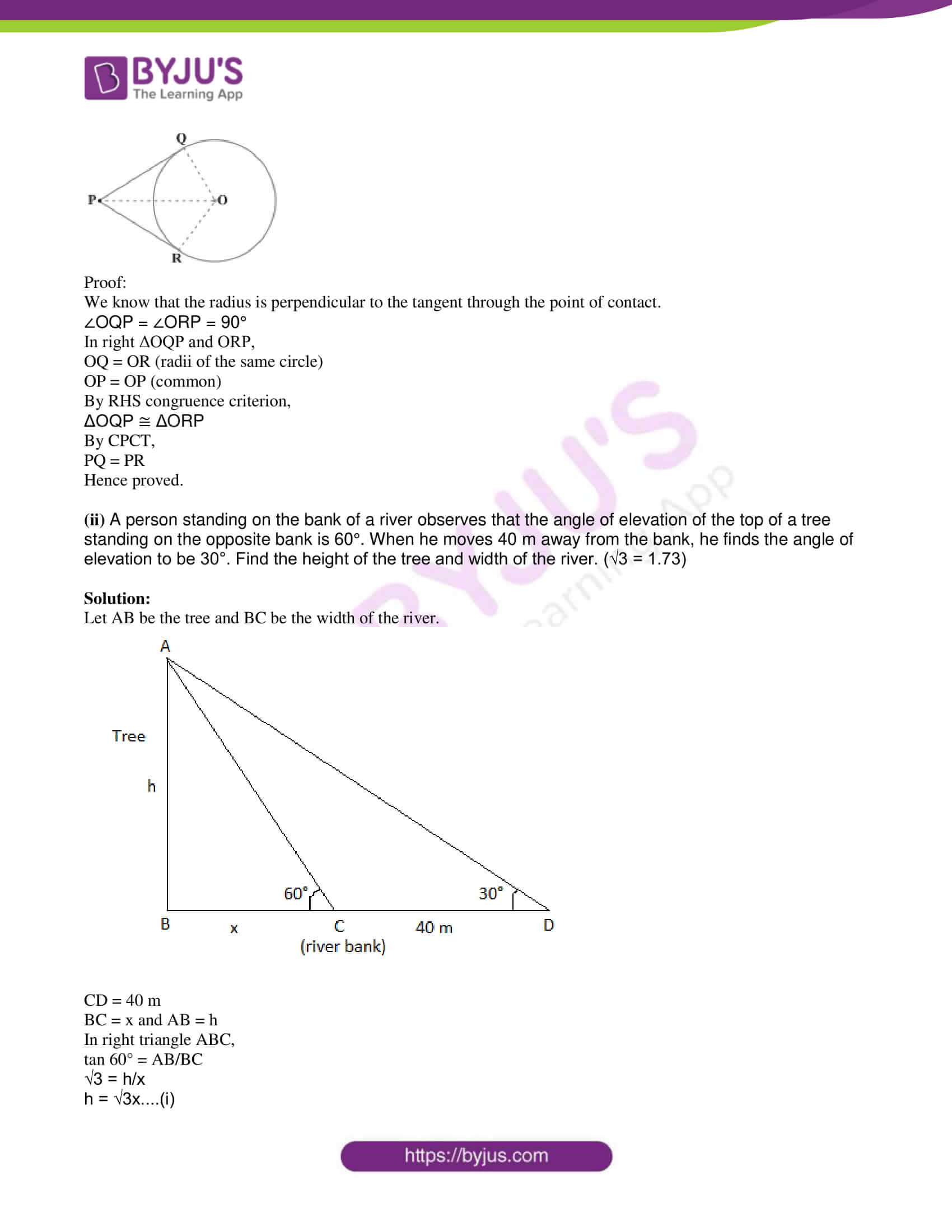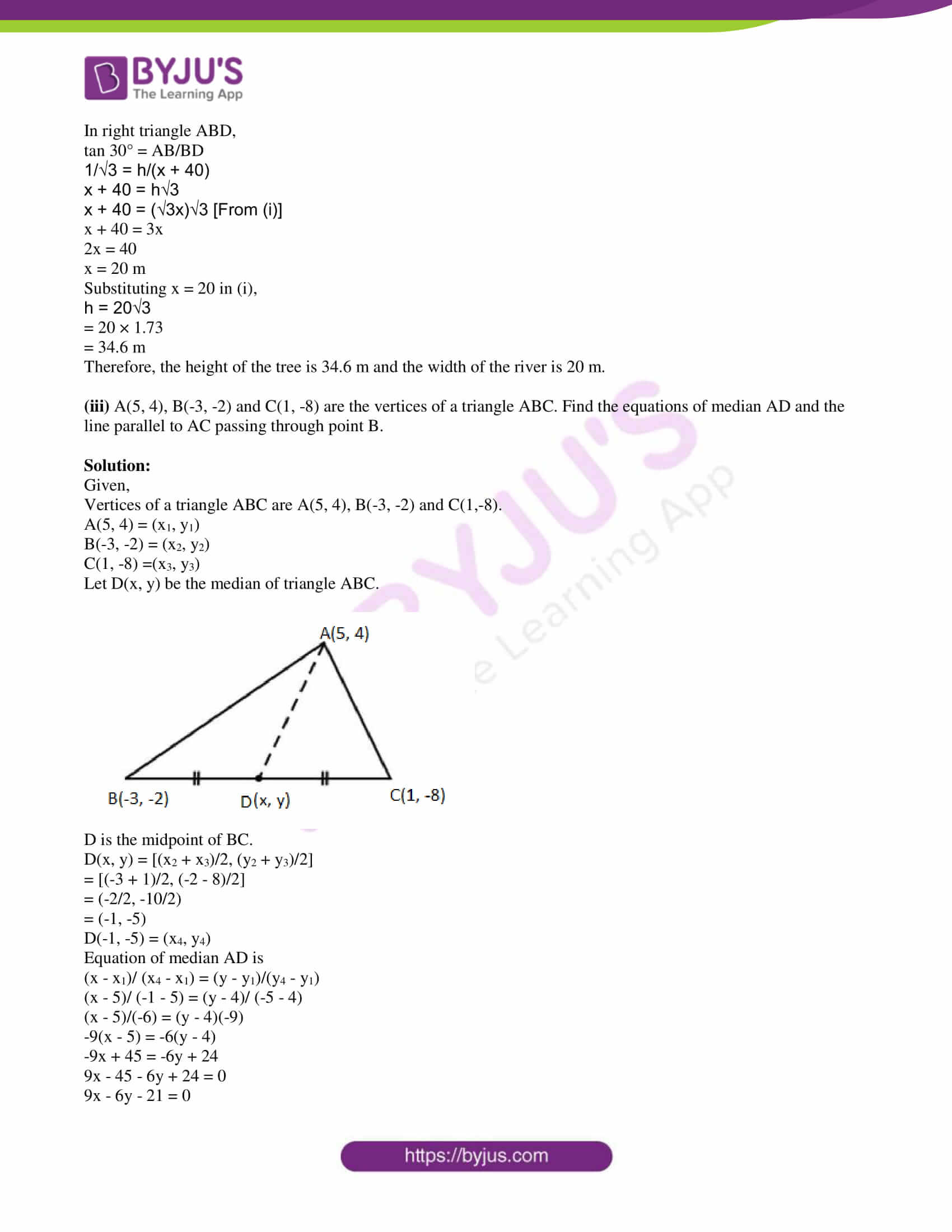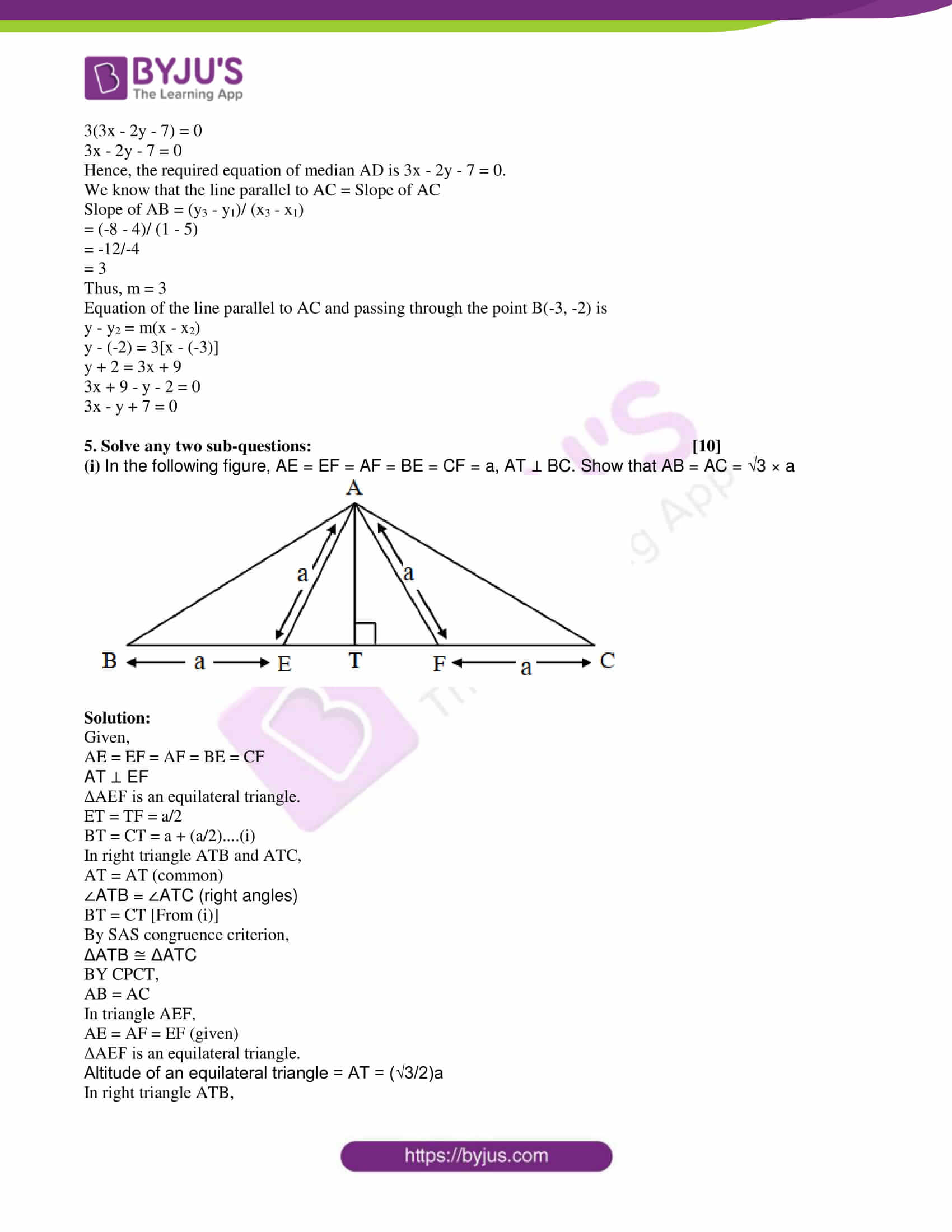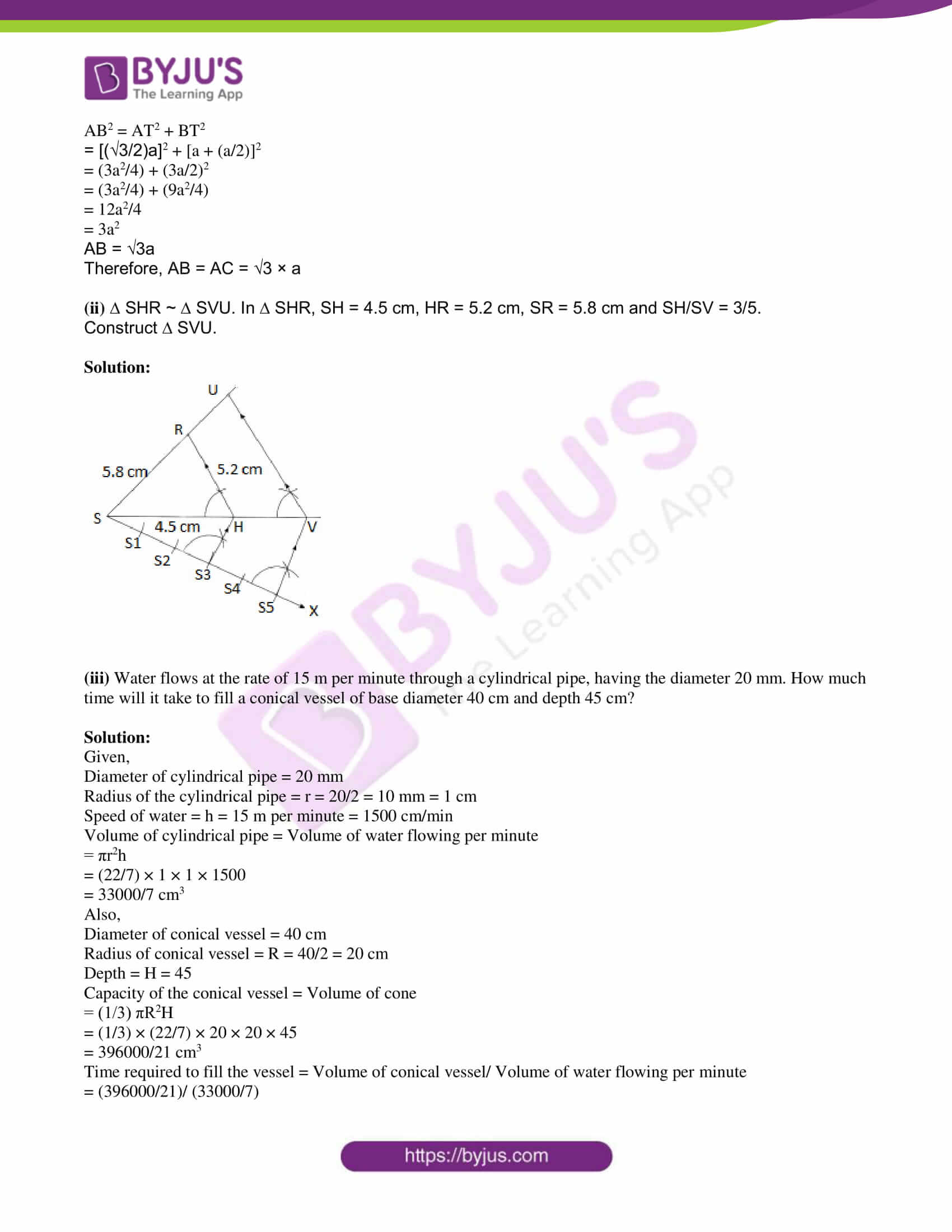PART – A

1. Solve any five sub-questions: 

(i) ∆DEF ~ ∆MNK. If DE = 2, MN = 5, then find the value of A(∆ DEF)/ A(∆ MNK).

Solution:

Given,

∆DEF ~ ∆MNK

DE = 2, MN = 5

We know that the ratio of areas of similar triangles is equal to squares of the ratio of their corresponding sides.

A(ΔDEF)/ A(ΔMNK) = DE2/MN2

= (2)2/(5)2

= 4/25

(ii) In the following figure, in ∆ABC, ∠B = 90°, ∠C = 60°, ∠A = 30°, AC = 16 cm. Find BC.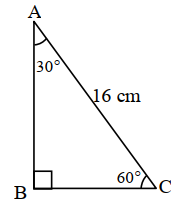Solution:

Given,

ΔABC is a 30° – 60° – 90° triangle.

Side opposite to 30° = BC

BC = (1/2) × Hypotenuse

= (1/2) × AC

= (1/2) × 16

= 8 cm

Therefore, BC = 8 cm

(iii) In the following figure, m(arc PMQ) = 110°, find ∠PQS.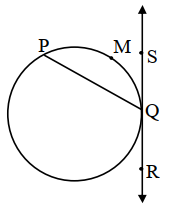Solution:

Given,

m(arc PMQ) = 110°

We know that the measure of the angle formed by the intersection of a chord and tangent of the circle equal to the half the angle made by the arc with the chord.

∠PQS = (½) × m(arc PMQ)

= (1/2) × 110°

= 55°

(iv) If the angle θ = -30°, find the value of cos θ.

Solution:

Given,

θ = -30°

We know that,

cos (-θ) = cos θ

cos θ = cos (-30°)

= cos 30°

= √3/2

(v) Find the slope of the line with inclination 60°.

Solution:

Given,

Inclination of line = θ = 60°

The slope of the line = tan θ

= tan 60°

= √3

(vi) Using Euler’s formula, find V if E = 10, F = 6.

Solution:

Given,

E = 10, F = 6

Using Euler’s formula,

F + V = E + 2

6 + V = 10 + 2

V = 12 – 6

V = 6

2. Solve any four sub-questions: 

(i) In the following figure, in ∆PQR, seg RS is the bisector of ∠PRQ. If PS = 9, SQ = 6, PR = 18, find QR.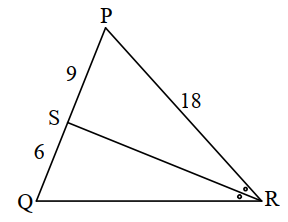Solution:

Given that, in triangle PQR, seg RS is the bisector of ∠PRQ.

PS = 9, SQ = 6, PR = 18

By the angle bisector property,

PR/QR = PS/SQ

18/QR = 9/6

⇒ QR = (18 × 6)/9

⇒ QR = 12

(ii) In the following figure, a tangent segment PA touching a circle in A and a secant PBC are shown. If AP = 12, BP = 9, find BC.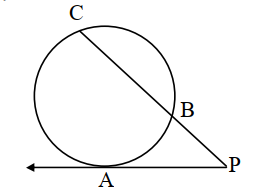Solution:

Given,

PA = tangent

PBC = secant

By the tangent – secant theorem,

PB × PC = PA2

9 × PC = (12)2 [given AP = 12, BP = 9]

PC = 144/9

PC = 16

Now,

PC = PB + BC

16 = 9 + BC

⇒ BC = 16 – 9

⇒ BC = 7

(iii) Draw an equilateral ∆ ABC with side 6.4 cm and construct its circumcircle.

Solution: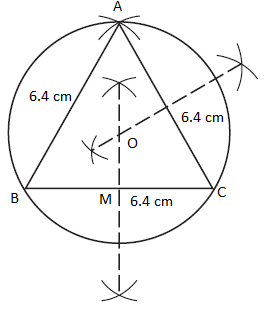(iv) For the angle in standard position if the initial arm rotates 130° in an anticlockwise direction, then state the quadrant in which the terminal arm lies. (Draw the Figure and write the answer.)

Solution:

Given,

The initial arm rotates 130° in the anticlockwise direction from the standard position.

The measure of angle 130° lies between 90° and 180°.

Hence, the terminal arm lies in quadrant II.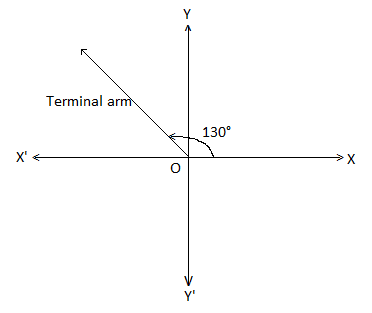(v) Find the area of the sector whose arc length and radius are 16 cm and 9 cm, respectively.

Solution:

Given,

Length of the arc = 16 cm

Radius = r = 9 cm

Area of sector = (r/2) × Length of arc

= (9/2) × 16

= 9 × 8

= 72 cm2

(vi) Find the surface area of a sphere of radius 1.4 cm. (π = 22/7)

Solution:

Given,

Radius of the sphere = r = 1.4 cm

The surface area of the sphere = 4πr2

= 4 × (22/7) × 1.4 × 1.4

= 24.64 cm2

Therefore, the surface area of the sphere is 24.64 cm2.

3. Solve any three sub-questions: 

(i) Adjacent sides of a parallelogram are 11 cm and 17 cm. If the length of one of its diagonals is 26 cm, find the length of the other.

Solution:

Given,

Adjacent sides of a parallelogram are 11 cm and 17 cm.

The length of one diagonal is 26 cm.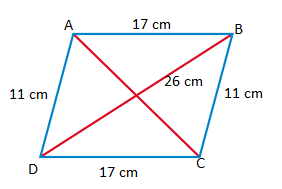AB = CD = 17 cm

BC = AD = 11 cm

BD = 26 cm

We know that,

Sum of squares of sides of a parallelogram = Sum of squares of its diagonals

AB2 + BC2 + CD2 + DA2 = AC2 + BD2

(17)2 + (11)2 + (17)2 + (11)2 = AC2 + (26)2

289 + 121 + 289 + 121 = AC2 + 676

AC2 = 820 – 676

AC2 = 144

AC = 12 cm

Therefore, the length of the other diagonal is 12 cm.

(ii) In the following figure, secants containing chords RS and PQ of a circle intersect each other in point A in the exterior of a circle. If m(arc PCR) = 26°, m(arc QDS) = 48°, then find:

a. m ∠PQR

b. m ∠SPQ

c. m ∠RAQ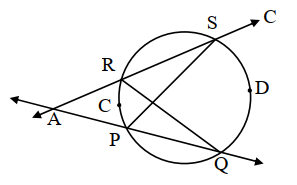Solution:

Given,

m(arc PCR) = 26°

m(arc QDS) = 48°

By the inscribed angle theorem,

a. ∠PQR = 1/2 × m(arc PCR)

= (1/2) × 26°

= 13°

∠PQR = ∠AQR = 13….(i)

b. ∠SPQ = 1/2 × m(arc QDS)

= (1/2) × 48°

= 24°

∠SPQ = 24°

c. In triangle AQR,

∠RAQ + ∠AQR = ∠SRQ (remote interior angle theorem)

∠SRQ = ∠SPQ (angles subtended by the same arc)

Therefore,

∠RAQ + ∠AQR = ∠SPQ

m∠RAQ = m∠SPQ – m∠AQR

= 24° – 13° [From (i) and (ii)]

= 11°

m∠RAQ = 11°

(iii) Draw a circle of radius 3.5 cm. Take any point K on it. Draw a tangent to the circle at K without using the centre of the circle.

Solution: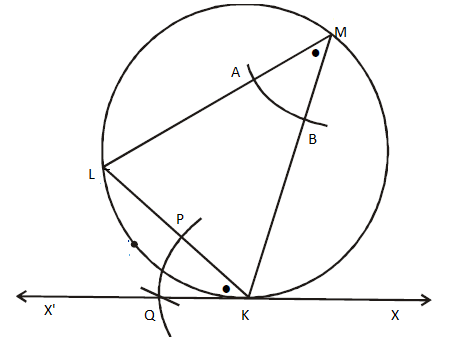Therefore, XKX’ is the required tangent to the circle with a radius of 3.5 cm.

(iv) If sec α = 2/√3, then find the value of (1 – cosec α)/ (1 + cosec α), where α is in IV quadrant.

Solution:

Given,

sec α = 2/√3

Thus, sec α = r/x = 2/√3

Let r = 2k and x = √3k

We know that,

r2 = x2 + y2

(2k)2 = (√3k)2 + y2

y2 = 4k2 – 3k2

y2 = k2

y = ±k

Given that α lies in quadrant IV.

Therefore, y = -k

cosec α = r/y = 2k/-k = -2

Now,

(1 – cosec α)/ (1 + cosec α)

= [1 – (-2)]/ [1 + (-2)]

= (1 + 2)/ (1 – 2)

= -3

(v) Write the equation of the line passing through the pair of points (2, 3) and (4, 7) in the form of y = mx + c.

Solution:

Let the given points be:

A(2, 3) = (x1, y1)

B(4, 7) = (x2, y2)

Equation of the line passing through the points (x1, y1) and (x2, y2) is:

(x – x1)/ (x2 – x1) = (y – y1)/ (y2 – y1)

(x – 2)/ (4 – 2) = (y – 3)/ (7 – 3)

(x – 2)/ 2 = (y – 3)/ 4

4(x – 2) = 2(y – 3)

4x – 8 = 2y – 6

4x – 8 – 2y + 6 = 0

4x – 2y – 2 = 0

2(2x – y – 1) = 0

2x – y – 1 = 0

y = 2x – 1

This is of the form y = mx + c [m = 2, c = -1]

Hence, the required equation of line is y = 2x – 1.

4. Solve any two sub-questions: 

(i) Prove that “The length of the two tangent segments to a circle drawn from an external point are equal”.

Solution:

Given,

PQ and PR are the tangents to the circle with centre O from an external point P.

To prove: PQ = PR

Construction:

Join OQ, OR and OP.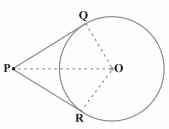Proof:

We know that the radius is perpendicular to the tangent through the point of contact.

∠OQP = ∠ORP = 90°

In right ΔOQP and ORP,

OQ = OR (radii of the same circle)

OP = OP (common)

By RHS congruence criterion,

ΔOQP ≅ ΔORP

By CPCT,

PQ = PR

Hence proved.

(ii) A person standing on the bank of a river observes that the angle of elevation of the top of a tree standing on the opposite bank is 60°. When he moves 40 m away from the bank, he finds the angle of elevation to be 30°. Find the height of the tree and width of the river. (√3 = 1.73)

Solution:

Let AB be the tree and BC be the width of the river.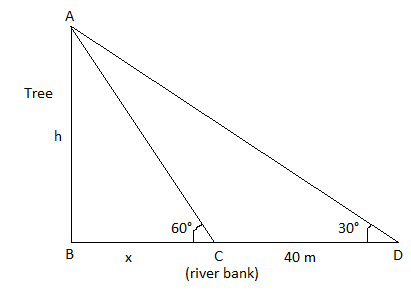CD = 40 m

BC = x and AB = h

In right triangle ABC,

tan 60° = AB/BC

√3 = h/x

h = √3x….(i)

In right triangle ABD,

tan 30° = AB/BD

1/√3 = h/(x + 40)

x + 40 = h√3

x + 40 = (√3x)√3 [From (i)]

x + 40 = 3x

2x = 40

x = 20 m

Substituting x = 20 in (i),

h = 20√3

= 20 × 1.73

= 34.6 m

Therefore, the height of the tree is 34.6 m and the width of the river is 20 m.

(iii) A(5, 4), B(-3, -2) and C(1, -8) are the vertices of a triangle ABC. Find the equations of median AD and the line parallel to AC passing through point B.

Solution:

Given,

Vertices of a triangle ABC are A(5, 4), B(-3, -2) and C(1,-8).

A(5, 4) = (x1, y1)

B(-3, -2) = (x2, y2)

C(1, -8) =(x3, y3)

Let D(x, y) be the median of triangle ABC.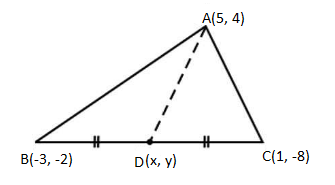D is the midpoint of BC.

D(x, y) = [(x2 + x3)/2, (y2 + y3)/2]

= [(-3 + 1)/2, (-2 – 8)/2]

= (-2/2, -10/2)

= (-1, -5)

D(-1, -5) = (x4, y4)

(x – x1)/ (x4 – x1) = (y – y1)/(y4 – y1)

(x – 5)/ (-1 – 5) = (y – 4)/ (-5 – 4)

(x – 5)/(-6) = (y – 4)(-9)

-9(x – 5) = -6(y – 4)

-9x + 45 = -6y + 24

9x – 45 – 6y + 24 = 0

9x – 6y – 21 = 0

3(3x – 2y – 7) = 0

3x – 2y – 7 = 0

Hence, the required equation of median AD is 3x – 2y – 7 = 0.

We know that the line parallel to AC = Slope of AC

Slope of AB = (y3 – y1)/ (x3 – x1)

= (-8 – 4)/ (1 – 5)

= -12/-4

= 3

Thus, m = 3

Equation of the line parallel to AC and passing through the point B(-3, -2) is

y – y2 = m(x – x2)

y – (-2) = 3[x – (-3)]

y + 2 = 3x + 9

3x + 9 – y – 2 = 0

3x – y + 7 = 0

5. Solve any two sub-questions: 

(i) In the following figure, AE = EF = AF = BE = CF = a, AT ⊥ BC. Show that AB = AC = √3 × a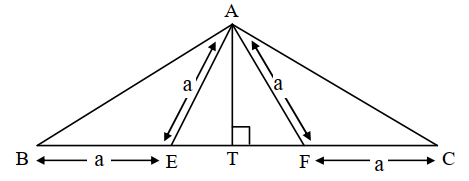Solution:

Given,

AE = EF = AF = BE = CF

AT ⊥ EF

ΔAEF is an equilateral triangle.

ET = TF = a/2

BT = CT = a + (a/2)….(i)

In right triangle ATB and ATC,

AT = AT (common)

∠ATB = ∠ATC (right angles)

BT = CT [From (i)]

By SAS congruence criterion,

ΔATB ≅ ΔATC

BY CPCT,

AB = AC

In triangle AEF,

AE = AF = EF (given)

ΔAEF is an equilateral triangle.

Altitude of an equilateral triangle = AT = (√3/2)a

In right triangle ATB,

AB2 = AT2 + BT2

= [(√3/2)a]2 + [a + (a/2)]2

= (3a2/4) + (3a/2)2

= (3a2/4) + (9a2/4)

= 12a2/4

= 3a2

AB = √3a

Therefore, AB = AC = √3 × a

(ii) ∆ SHR ~ ∆ SVU. In ∆ SHR, SH = 4.5 cm, HR = 5.2 cm, SR = 5.8 cm and SH/SV = 3/5.

Construct ∆ SVU.

Solution: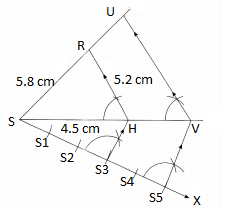(iii) Water flows at the rate of 15 m per minute through a cylindrical pipe, having the diameter 20 mm. How much time will it take to fill a conical vessel of base diameter 40 cm and depth 45 cm?

Solution:

Given,

Diameter of cylindrical pipe = 20 mm

Radius of the cylindrical pipe = r = 20/2 = 10 mm = 1 cm

Speed of water = h = 15 m per minute = 1500 cm/min

Volume of cylindrical pipe = Volume of water flowing per minute

= πr2h

= (22/7) × 1 × 1 × 1500

= 33000/7 cm3

Also,

Diameter of conical vessel = 40 cm

Radius of conical vessel = R = 40/2 = 20 cm

Depth = H = 45

Capacity of the conical vessel = Volume of cone

= (1/3) πR2H

= (1/3) × (22/7) × 20 × 20 × 45

= 396000/21 cm3

Time required to fill the vessel = Volume of conical vessel/ Volume of water flowing per minute

= (396000/21)/ (33000/7)

= (396000 × 7)/ (21 × 33000)

= 4

Hence, the time required to fill the conical vessel is 4 min.